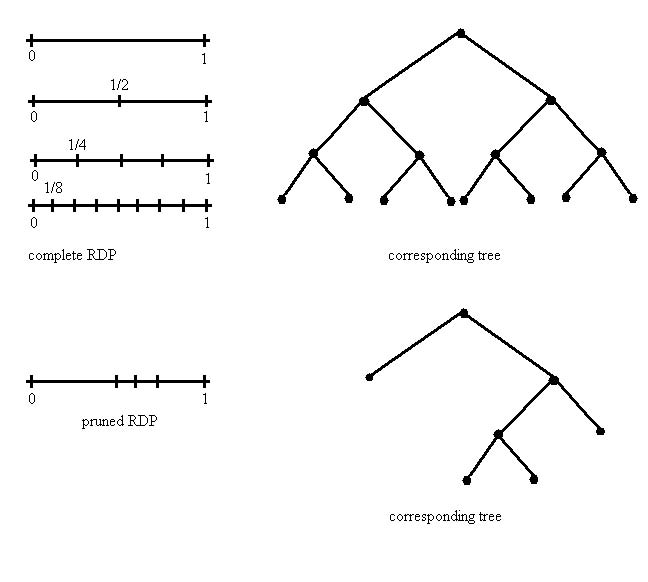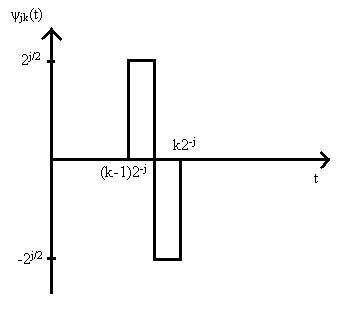# 0.16 Nonlinear approximation and wavelet analysis  (Page 2/2)

 Page 2 / 2

Suppose $f$ is piecewise Lipschitz and ${f}_{k}$ ia a piecewise constant.

$|f\left(t\right)-{f}_{k}\left(t\right)|\approx \Delta$

where $\Delta$ is a constant equal to average of $f$ on right and left side of discontinuity in this interval.

$⇒||f-{f}_{k}{||}_{{L}_{2}}^{2}=O\left({k}^{-1}\right)$

where ${k}^{-1}$ is the width of the interval. Notice this rate is quite slow.

This problem naturally suggests the following remedy: use very small intervals near discontinuities and larger intervals insmooth regions. Specifically, suppose we use intervals of width ${k}^{-2\alpha }$ to contain the discontinuities and the intervals ofwidth ${k}^{-1}$ elsewhere. Then accordingly piecewise polynomial approximation ${\stackrel{˜}{f}}_{k}$ satisfies

$||f-{\stackrel{˜}{f}}_{k}{||}_{{L}_{2}}^{2}=O\left({k}^{-2\alpha }\right).$

We can accomplish this need for "adaptive resolution" or "multiresolution" using recursive partitions and trees.

## Recursive dyadic partitions

We discussed this idea already in our examination of classification trees. Here is the basic idea again, graphically.Complete and pruned RDP along with their correspnding tree structures.

Consider a function $f\in {B}^{\alpha }\left({C}_{\alpha }\right)$ that contains no more than m points of discontinuity, and is ${H}^{\alpha }\left({C}_{\alpha }\right)$ away from these points.

Lemma

Consider a complete RDP with n intervals, then there exists anassociated pruned RDP with $O\left(klogn\right)$ intervals, such that an associated piecewise degree $⌈\alpha ⌉$ polynomial approximation $\stackrel{˜}{\left(}{f\right)}_{k}$ , has a squared approximation error of $O\left(min\left({k}^{-2\alpha },{n}^{-1}\right)\right)$ .

Assume $n>k>m$ . Divide $\left[0,1\right]$ into $k$ intervals. If $f$ is smooth on a particular interval $I$ , then

$|f\left(t\right)-{\stackrel{˜}{f}}_{k}\left(t\right)|=O\left({k}^{-2\alpha }\right)\forall t\in I.$

In intervals that contain a discontinuity, recursively subdivide into two until the discontinuity is contained in an interval ofwidth ${n}^{-1}$ . This process results in at most $lo{g}_{2}n$ addition subintervals per discontinuity, and the squared approximationerror is $O\left(k-2\alpha \right)$ on all of them accept the $m$ intervals of width ${n}^{-1}$ containing the discontinuities where the error is $O\left(1\right)$ at each point.

Thus, the overall squared ${L}_{2}$ norm is

$||f-{\stackrel{˜}{f}}_{k}{||}_{{L}_{2}}^{2}=O\left(min\left({k}^{-2\alpha },{n}^{-1}\right)\right)$

and there are at most $k+lo{g}_{2}n$ intervals in the partition. Since k>m, we can upperbound the number of intervals by $2klo{g}_{2}n$ .

Note that if the initial complete RDP has $n\approx {k}^{2\alpha }$ intervals, then the squared error is $O\left({k}^{-2\alpha }\right)$ .

Thus, we only incur a factor of $2\alpha logk$ additional leafs and achieve the same overall approximation error as in the ${H}^{\alpha }\left({C}_{\alpha }\right)$ case. We will see that this is a small price to pay in order to handle not only smooth functions, but alsopiecewise smooth functions.

## Wavelet approximations

Let $f\in {L}^{2}\left(\left[0,1\right]\right)$ ; $\int {f}^{2}\left(t\right)dt<\infty$ .

A wavelet approximation is a series of the form

$f={c}_{o}+\sum _{j\ge 0}\sum _{k=1}^{{2}^{j}}{\psi }_{j,k}$

where ${c}_{o}$ is a constant $\left({c}_{o}={\int }_{0}^{1}f\left(t\right)dt\right)$ ,

$={\int }_{0}^{1}f\left(t\right){\psi }_{j,k}\left(t\right)dt$

and the basis functions ${\psi }_{j,k}$ are orthonormal, oscillatory signals, each with an associated scale ${2}^{-j}$ and position $k{2}^{-j}$ . ${\psi }_{j,k}$ is called the wavelet at scale ${2}^{-j}$ and position $k{2}^{-j}$ .

## Haar wavelets

${\psi }_{j,k}\left(t\right)={2}^{j/2}\left({\mathbf{1}}_{\left\{t\in \left[{2}^{-j}\left(k-1\right),{2}^{-j}\left(k-1/2\right)\right]\right\}}-{\mathbf{1}}_{\left\{t\in \left[{2}^{-j}\left(k-1/2\right),{2}^{-j}k\right]\right\}}\right)$Haar Wavelet
${\int }_{0}^{1}{\psi }_{j,k}\left(t\right)dt=0$
${\int }_{0}^{1}{\psi }_{j,k}^{2}\left(t\right)dt={\int }_{\left(k-1\right){2}^{-j}}^{k{2}^{-j}}{2}^{j}dt=1$
${\int }_{0}^{1}{\psi }_{j,k}\left(t\right){\psi }_{l,m}\left(t\right)dt={\delta }_{j,l}.{\delta }_{k,m}$
If $f$ is constant on $\left[{2}^{-j}\left(k-1\right),{2}^{-j}k\right]$ , then
$\int f{\psi }_{j,k}\left(t\right)=0.$

Suppose $f$ is piecewise constant with at most $m$ discontinuities. Let

${f}_{J}={c}_{o}+\sum _{j=0}^{J-1}\sum _{k=1}^{{2}^{j}}{\psi }_{j,k}.$

Then, ${f}_{J}$ has at most $mJ$ non-zero wavelet coefficients; i.e., $=0$ for all but $mJ$ terms, since at most one Haar Wavelet at each scale senses each point of discontinuity. Said another way, allbut at most $m$ of the wavelets at each scale have support over constant regions of $f$ .

${f}_{J}$ itself will be piecewise constant with discontinuities only possible occurring at end points of the intervals $\left[{2}^{-J}\left(k-1\right),{2}^{-J}k\right]$ . Therefore, in this case

$||f-{f}_{J}{||}_{{L}_{2}}^{2}=O\left({2}^{-J}\right).$

Daubechies wavelets are the extension of the Haar wavelet idea. Haar wavelets have one "vanishing moment":

${\int }_{0}^{1}{\psi }_{j,k}=0.$

Daubechies wavelets are "smoother" basis functions with extra vanishing moments. The Daubechies- $N$ wavelet has $N$ vanishing moments.

${\int }_{0}^{1}{t}^{l}{\psi }_{j,k}dt=0forl=0,1,...,N-1.$

The Daubechies-1 wavelet is just the Haar case.

If $f$ is a piecewise degree $\le N$ polynomial with at most m pieces, then using the Daubechies- $N$ wavelet system.

$||f-{f}_{J}{||}_{{L}_{2}}^{2}=O\left({2}^{-J}\right);$

and

${f}_{J}\left(t\right)={c}_{o}+\sum _{j=0}^{J-1}\sum _{k=1}^{{2}^{j}}{\psi }_{j,k}\left(t\right)$

has at most $O\left(mJ\right)$ non-zero wavelet coefficients. ${f}_{J}$ is called the Discrete Wavelet Transform (DWT) approximation of $f$ . The key idea is the same as we saw with trees.

## Sampled data

We can also use DWT's to analyze and represent discrete, sampled functions. Suppose,

$\underline{f}=\left[f\left(1/n\right),f\left(2/n\right),...,f\left(n/n\right)\right]$

then we can write $\underline{f}$ as

$\underline{f}={c}_{o}+\sum _{j=0}^{lo{g}_{2}n-1}\sum _{k=1}^{{2}^{j}}<\underline{f},{\underline{\psi }}_{j,k}>{\underline{\psi }}_{j,k}$

where

${\underline{\psi }}_{j,k}=\left[{\psi }_{j,k}\left(1\right),{\psi }_{j,k}\left(2\right),...,{\psi }_{j,k}\left(n\right)\right]$

is a discrete time analog of the continuous time wavelets we considered before. In particular,

$\sum _{i=1}^{n}{i}^{l}{\psi }_{j,k}\left(i\right)=0,l=0,1,...,N-1$

for the Daubechies- $N$ discrete wavelets.

$<\underline{f},{\underline{\psi }}_{j,k}>={\underline{f}}^{T}{\underline{\psi }}_{j,k}$

Thus, we also have an analogous approximation result: If $\underline{f}$ are samples from a piecewise degree $\le N$ polynomial function with a finite number $m$ of discontinuities, then $\underline{f}$ has $O\left(mJ\right)$ non-zero wavelet coefficients.

## ApproximatingFunctions with wavelets

Suppose $f\in {B}^{\alpha }\left({C}_{\alpha }\right)$ and has a finite number of discontinuities. Let ${f}_{p}$ denote piecewise degree- $N\left(N=⌈\alpha ⌉\right)$ polynomial approximation to $f$ with $O\left(k\right)$ pieces; a uniform partition into $k$ equal length intervals followed by addition splits at the points of discontinuity.

Then

$|f\left(t\right)-{f}_{p}\left(t\right){|}^{2}=O\left({k}^{\left(}-2\alpha \right)\right)\forall t\in \left[0,1\right]$
$⇒|f\left(i/n\right)-{f}_{p}{\left(i/n\right)|}^{2}=O\left({k}^{-2\alpha }\right)i=1,...,n$
$⇒1/n||\underline{f}-{\underline{f}}_{p}{||}_{{L}_{2}}^{2}=O\left({k}^{-2\alpha }\right)\right)$

and ${\underline{f}}_{p}$ has $O\left(klo{g}_{2}n\right)$ non-zero coefficients according to our previous analysis.

## Wavelets in 2-d

Suppose $f$ is a 2-D image that is piecewise polynomial:

A pruned RDP of $k$ squares decorated with polyfits gives

$||f-{f}_{k}{||}_{{L}_{2}}^{2}=O\left({k}^{-1}\right).$

Let $\underline{f}{=\left[f\left(i/k,j/k\right)}_{i,j=1}^{n}$ sample range.

${f}_{n}\left(t\right)=\sum _{i,j=1}^{k}f\left(i/k,j/kk\right){\mathbf{1}}_{\left\{t\in \left[i-1/k,i/k\right)x\left[j-1/k,j/k\right)\right\}}$

then

$||f-{f}_{n}{||}_{{L}_{2}}^{2}=O\left({k}^{-1}\right)$

$O\left(1\right)$ error on $k$ of the ${k}^{2}$ pixels, near zero elsewhere. The DWT of $\underline{f}$ has $O\left(k\right)$ non-zero wavelet coefficients. $O\left({2}^{j}\right)$ at scale ${2}^{-j},j=0,1,...,logn.$

#### Questions & Answers

Is there any normative that regulates the use of silver nanoparticles?
Damian Reply
what king of growth are you checking .?
Renato
What fields keep nano created devices from performing or assimulating ? Magnetic fields ? Are do they assimilate ?
Stoney Reply
why we need to study biomolecules, molecular biology in nanotechnology?
Adin Reply
?
Kyle
yes I'm doing my masters in nanotechnology, we are being studying all these domains as well..
Adin
why?
Adin
what school?
Kyle
biomolecules are e building blocks of every organics and inorganic materials.
Joe
anyone know any internet site where one can find nanotechnology papers?
Damian Reply
research.net
kanaga
sciencedirect big data base
Ernesto
Introduction about quantum dots in nanotechnology
Praveena Reply
what does nano mean?
Anassong Reply
nano basically means 10^(-9). nanometer is a unit to measure length.
Bharti
do you think it's worthwhile in the long term to study the effects and possibilities of nanotechnology on viral treatment?
Damian Reply
absolutely yes
Daniel
how to know photocatalytic properties of tio2 nanoparticles...what to do now
Akash Reply
it is a goid question and i want to know the answer as well
Maciej
characteristics of micro business
Abigail
for teaching engĺish at school how nano technology help us
Anassong
Do somebody tell me a best nano engineering book for beginners?
s. Reply
there is no specific books for beginners but there is book called principle of nanotechnology
NANO
what is fullerene does it is used to make bukky balls
Devang Reply
are you nano engineer ?
s.
fullerene is a bucky ball aka Carbon 60 molecule. It was name by the architect Fuller. He design the geodesic dome. it resembles a soccer ball.
Tarell
what is the actual application of fullerenes nowadays?
Damian
That is a great question Damian. best way to answer that question is to Google it. there are hundreds of applications for buck minister fullerenes, from medical to aerospace. you can also find plenty of research papers that will give you great detail on the potential applications of fullerenes.
Tarell
what is the Synthesis, properties,and applications of carbon nano chemistry
Abhijith Reply
Mostly, they use nano carbon for electronics and for materials to be strengthened.
Virgil
is Bucky paper clear?
CYNTHIA
carbon nanotubes has various application in fuel cells membrane, current research on cancer drug,and in electronics MEMS and NEMS etc
NANO
so some one know about replacing silicon atom with phosphorous in semiconductors device?
s. Reply
Yeah, it is a pain to say the least. You basically have to heat the substarte up to around 1000 degrees celcius then pass phosphene gas over top of it, which is explosive and toxic by the way, under very low pressure.
Harper
Do you know which machine is used to that process?
s.
how to fabricate graphene ink ?
SUYASH Reply
for screen printed electrodes ?
SUYASH
What is lattice structure?
s. Reply
of graphene you mean?
Ebrahim
or in general
Ebrahim
in general
s.
Graphene has a hexagonal structure
tahir
On having this app for quite a bit time, Haven't realised there's a chat room in it.
Cied
what is biological synthesis of nanoparticles
Sanket Reply
how did you get the value of 2000N.What calculations are needed to arrive at it
Smarajit Reply
Privacy Information Security Software Version 1.1a
Good
Got questions? Join the online conversation and get instant answers!
Jobilize.com Reply

### Read also:

#### Get the best Algebra and trigonometry course in your pocket!

Source:  OpenStax, Statistical learning theory. OpenStax CNX. Apr 10, 2009 Download for free at http://cnx.org/content/col10532/1.3
Google Play and the Google Play logo are trademarks of Google Inc.

Notification Switch

Would you like to follow the 'Statistical learning theory' conversation and receive update notifications?ByBy David Martin